# CLASS 10 MATH NCERT SOLUTIONS FOR CHAPTER – 3 PAIR OF LINEAR EQUATIONS IN TWO VARIABLES EX – 3.5

## Pair of Linear Equations in Two Variables

Question 1.
Which of the following pairs of linear equations has unique solution, no solution or infinitely many solutions. In case there is unique solution, find it by using cross-multiplication method.

(i) x – 3y – 3 = 0, 3x – 9y – 2 = 0
(ii) 2x + y = 5, 3x + 2y = 8
(iii) 3x – 5y = 20, 6x – 10y = 40
(iv) x – 3y – 7 = 0, 3x – 3y – 15 = 0

Solution:

(i) x – 3y – 3 = 0  ….(1)
3x – 9y – 2 = 0 …..(2)Therefore, the pair of linear equations has no solution.

(ii)
2x + y – 5 = 0  …..(1)
3x + 2y – 8 = 0 …..(2)Therefore, the pair of linear equations has a unique solution.
Now by cross-multiplication method, we have: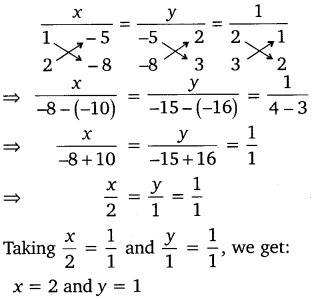Hence, the required solution is x and y = 1.

(iii)
3x – 5y = 20 …….(1)
6x – 10y – 40 = 0 …..(2)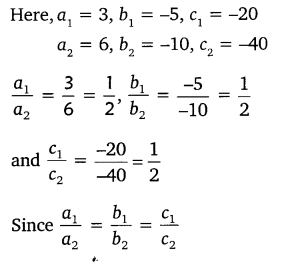Therefore, the pair of linear equations has infinitely many solutions.

(iv) x – 3y – 7 = 0 …(1)
3x- 3y- 15 = 0     …(2)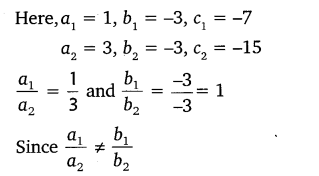Therefore, the pair of linear equations has a unique solution.
Now by cross-multiplication method, we have: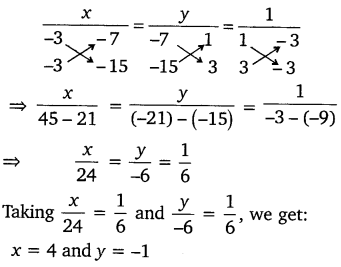Hence, the required solution is x = 4 and y = -1.

Question 2.

(i) For which values of a and b does the following pair of linear equations have an infinite number of solutions?
2x + 3y = 7
(a – b)x + (a + b)y = 3a + b – 2

(ii) For which value of k will the following pair of linear equations have no solution?
3x + y = 1
(2k – 1)x + (k – 1)y = (2k + 1)

Solution:
(i)
We have:
2x + 3y – 7 = 0
(a – b)x + (a + b)y – (3a + b -2) = 0
Here, a1 = 2, b1 = 3, c1 = -7
a2 = (a – b), b2 = (a + b), c2 = -(3a + b – 2)
We know that for a pair of linear equations to have infinite numbers of solutions(ii) We have: 3x + y – 1 = 0
(2k – 1)x + (k – 1)y – (2k + 1) = 0
Here, a1 = 3, b1 = 1, c1 = -1
a2 = 2k – 1, b2 = k – 1 , c1 = -(2k +1)
Since the pair of linear equations has no solution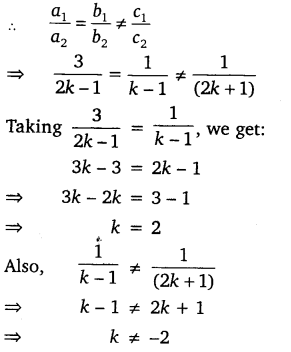Thus, the given pair of linear equations has no solution when k = 2.

Question 3.
Solve the following pair of linear equations by the substitution and cross-multiplication methods:
8x + 5y = 9, 3x + 2y = 4

Solution:
We have:  8x + 5y = 9 ……(i)
3x + 2y = 4 …..(ii)

Substitution method:

Multiplying equation (i) by 2 and equation (ii) by 5 and then subtracting the results, we get:
16x – 15x = 18-20
⇒ x = -2
Substituting x = -2 in equation (i), we get:
8(-2) + 5y = 9
⇒  -16 + 5y = 9
⇒   5y  = 25
⇒ y = 5
Thus, the required solution is x = -2 and y = 5.

Cross multiplication method:
8x + 5y – 9 = 0
3x + 2y – 4 = 0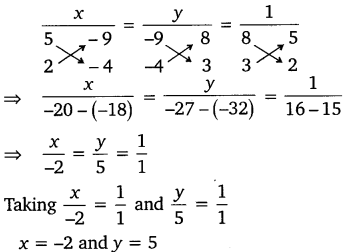Thus, the required solution is x = -2 and y = 5.

Question 4.
Form the pair of linear equations in the following problems and find their solutions (if they exist) by any algebraic method:

(i) A part of monthly hostel charges is fixed and the remaining depends on the number of days one has taken food in the mess. When a student A takes food for 20 days she has to pay ₹ 1000 as hostel charges, whereas a student B, who takes food for 26 days, pays ₹ 1180 as hostel charges. Find the fixed charges and the cost of food per day.

(ii) A fraction becomes$\frac { 1 }{ 3 }$ when 1 is subtracted from the numerator and it becomes$\frac { 1 }{ 4 }$ when 8 is added to its denominator. Find the fraction.

(iii) Yash scored 40 marks in a test, getting 3 marks for each right answer and losing 1 mark for each wrong answer. Had 4 marks been awarded for each correct answer and 2 marks been deducted for each incorrect answer, then Yash would have scored 50 marks. How many questions were there in the test?

(iv) Places A and B are 100 km apart on a highway. One car starts from A and another from B at the same time. If the cars travel in the same direction at different speeds, they meet in 5 hours. If they travel towards each other, they meet in 1 hour. What are the speeds of the two cars?

(v) The area of a rectangle gets reduced by 9 square units, if its length is reduced by units and breadth is increased by 3 units. If we increase the length by 3 units and the breadth by 2 units, the area increases by 67 square units. Find the dimensions of the rectangle.

Solution:

[Note: These problems can be solved by any algebraic method. Here, we have solved by the elimination method.]

(i) Let the fixed monthly charges of hostel be ₹ x and cost of food for one day be ₹ y.
Then according to the question, we have:
Total charges for student A:
x + 20y = 1000   …(1)
Total charges for student B:
x + 26y = 1180   …(2)
Subtracting equation (1) from equation (2), we get: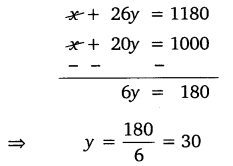Substituting the value ofy in equation (1), we get:
x + 20 x 30 = 1000
⇒ x = 1000 – 20 x 30
= 1000 – 600 = 400
Thus, the fixed monthly charges are ? 400 and cost of food per day is ? 30.

(ii) Let the fraction be$\frac { x }{ y }$.
Then according to the question, we have: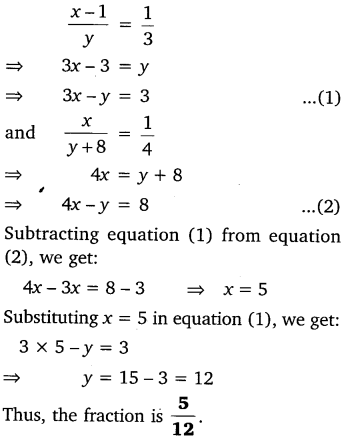(iii) Let the number of right answers and wrong answers be x and y respectively
Then according to the question, we have:
3x – y = 40        …(1)
and 4x – 2y = 50    …(2)
Multiplying equation (1) by 2 and then subtracting the result from (2), we get:
4x – 6x = 50 – 80
⇒  – 2x = -30
⇒ x = 15
Substituting x = 15 in equation (1), we get:
3  x 15 -y = 40
⇒  45 -y = 40
⇒  y   = 5
Thus, the total number of questions in the test = x + y
= 15 + 5 = 20.

(iv) Let the speed of first car be x km/h and that of second car be y km/h.
Case I: When both the cars travel in the same direction
Distance travelled by first car = 5x km
Distance travelled by second car = 5y km
Distance between both the cars
5x – 5y = 100  [Given]
⇒  x- y = 20   …(1)
Case II: When both the cars travel in opposite directions
Distance between both the cars
= x + y = 100   …(2) [Given]
Adding equations (1) and (2), we get:
2x= 120
⇒ x = 60
Substituting x = 60 in equation (2), we get:
60 + y = 100
⇒    y = 100 – 60 = 40
Thus, the speeds of the cars are 60 km/h and 40 km/h.

(v) Let the length and breadth of a rectangle be x units and y units respectively.
Then the area of the rectangle = xy sq. units.

Case 1: When the length is reduced by 5 units and breadth is increased by 3 units
New length = (x – 5) units
New breadth = (y + 3) units
∴ New area = (x – 5) (y + 3) sq.
units According to the question, we have:
xy – (x – 5) (y + 3) =9
⇒  xy – (xy + 3x – 5y – 15) = 9
⇒  xy-xy-3x + 5y+15=9
⇒ -3x + 5y = -6 …(1)

Case 2: When the length is increased by 3 units and breadth is increased by 2 units
New length = (x + 3) units New breadth
New breadth (y + 2) units
∴  New area = (x + 3) (y + 2) sq units
According to the question, we have:
(x+3) (y+2)- xy =67
⇒ xy + 3y + 2x + 6 – xy = 67
⇒ 2x + 3y = 61 …(2)
Multiplying equation (1) by 2 and (2) by 3 and then adding the results, we get:
10y + 9y = -12+ 183
⇒ 19y =171
⇒ y=9
Substituting y = 9 in equation (1), we get:
-3x + 5 x 9 = -6
⇒ -3x = -6-45
⇒ x = 17
Thus, the length of the rectangle is 17 units and its breadth is 9 units.